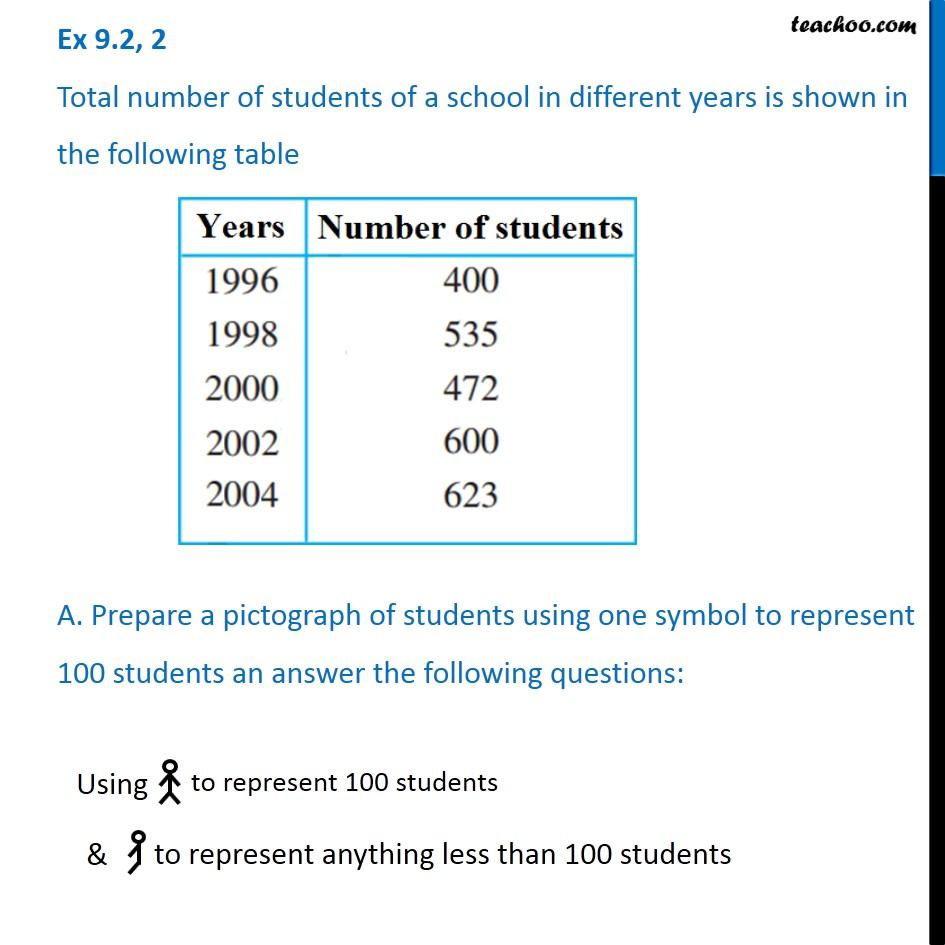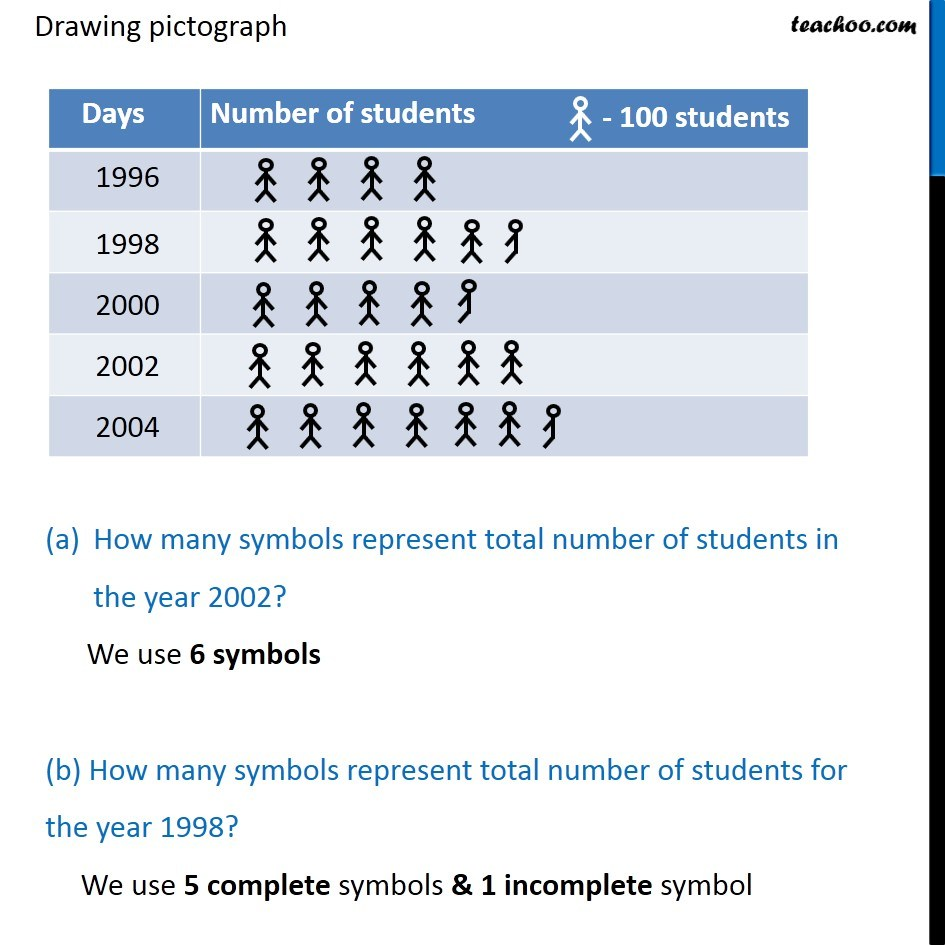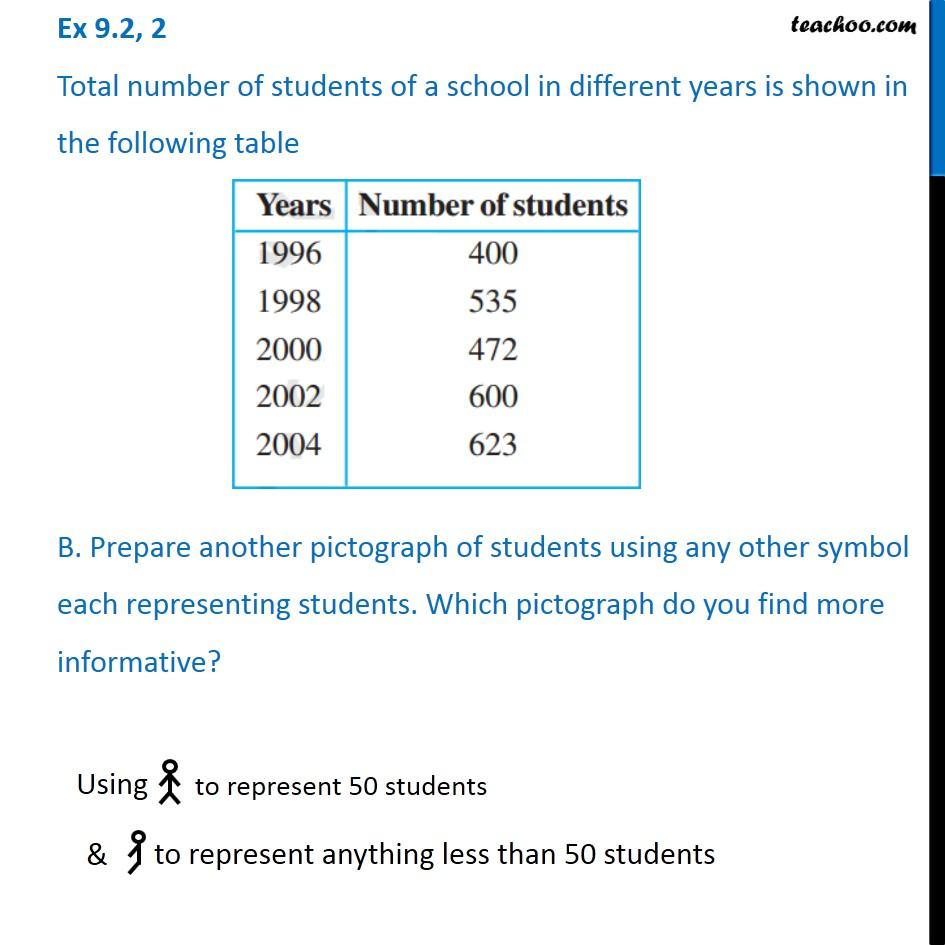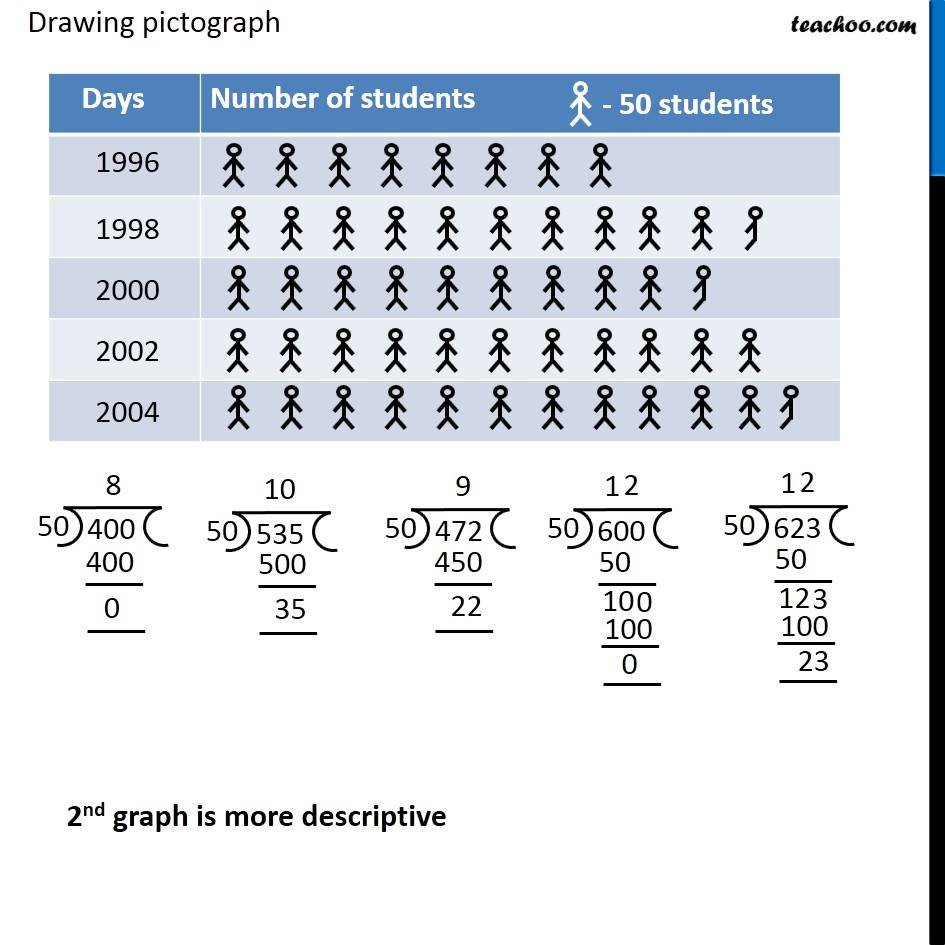Subscribe to our Youtube Channel - https://you.tube/teachoo

1. Chapter 9 Class 6 Data Handling
2. Serial order wise
3. Ex 9.2

Transcript

Ex 9.2, 2 Total number of students of a school in different years is shown in the following table A. Prepare a pictograph of students using one symbol to represent 100 students an answer the following questions: Using to represent 100 students to represent anything less than 100 students Drawing pictograph How many symbols represent total number of students in the year 2002? We use 6 symbols (b) How many symbols represent total number of students for the year 1998? We use 5 complete symbols & 1 incomplete symbol Drawing pictograph How many symbols represent total number of students in the year 2002? We use 6 symbols (b) How many symbols represent total number of students for the year 1998? We use 5 complete symbols & 1 incomplete symbol Ex 9.2, 2 Total number of students of a school in different years is shown in the following table B. Prepare another pictograph of students using any other symbol each representing students. Which pictograph do you find more informative? Drawing pictograph 2nd graph is more descriptive

Ex 9.2